# Simple Cost Calculators

Lets say the calculator is about calculating the cost of building a video campaign. Here are 4 sample questions: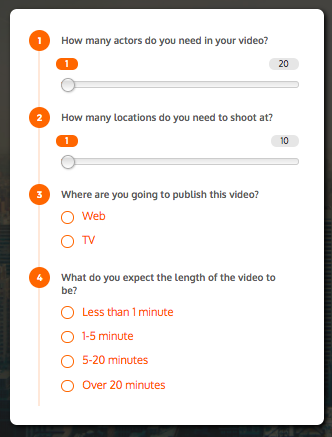The first two questions are simple sliders. So the user will use the sliders to select a value. Pretty simple.The third question is about where the video needs to be posted. If the video needs to be posted on the web, then let's say it needs \$5000 worth of editing. So, we will add \$5000 as the "value" for web. For TV, we have assumed that it will require \$20000 worth of editing.Question 4 is about the length of the video. Here, we are assuming that a 20 minute video takes 4 times the effort of a <1 minute video.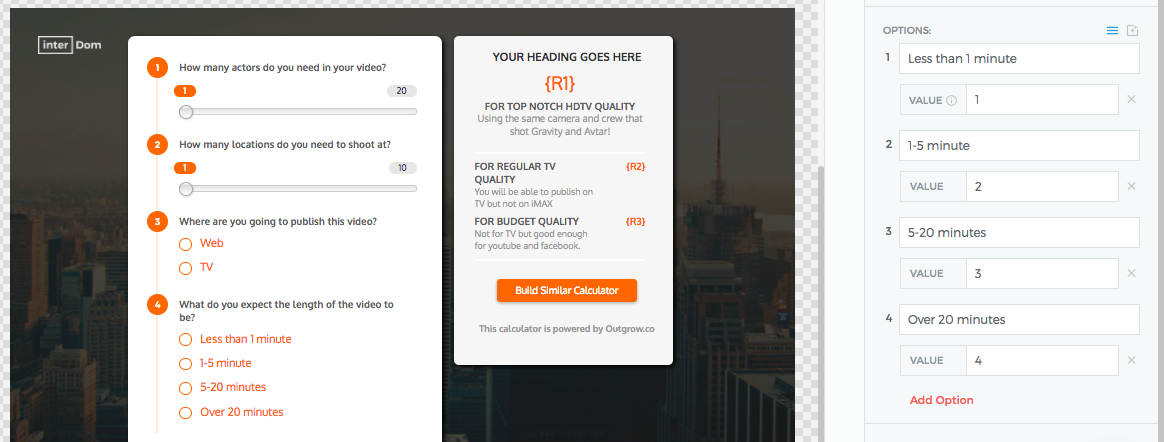Now that we have the questions out of the way, we have to build the formula. Handling the first 2 questions is easy.

For Q1, say the cost of each actor is \$500. So we just multiply the input of Q1 (number of actors) with the cost of each calculator. We create a similar formula for Q2 as well.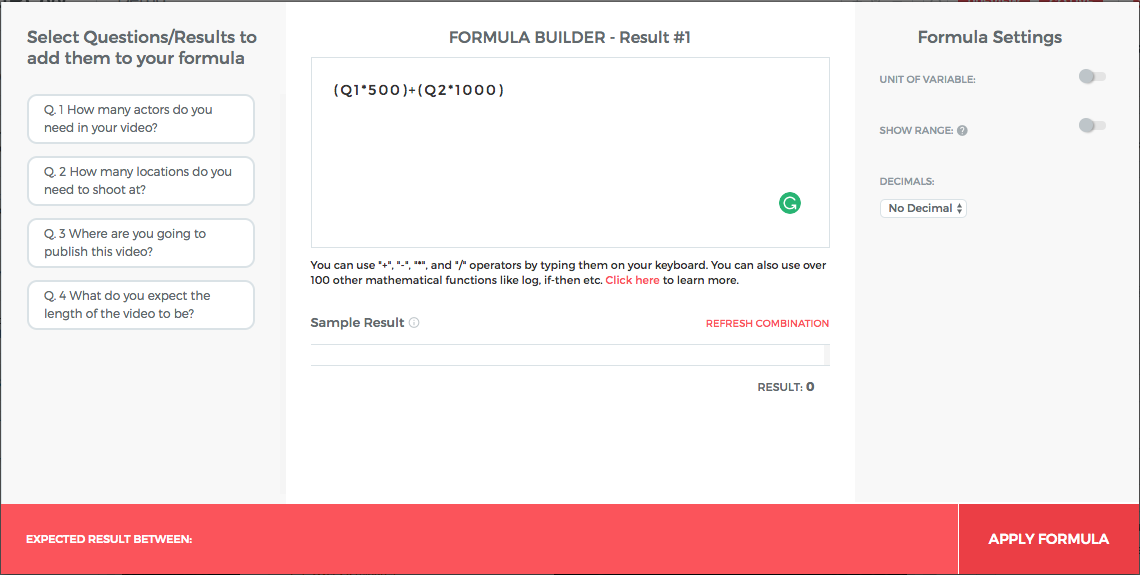To handle for Q3, we will just simply add the editing cost for web or for TV which has already been assigned while creating the question.

For Q4 (length of the video), we have assumed that the length of the video will determine the overall effort put in by the actors and editors. So we can simply multiply the input for Q4 with the rest of the formula.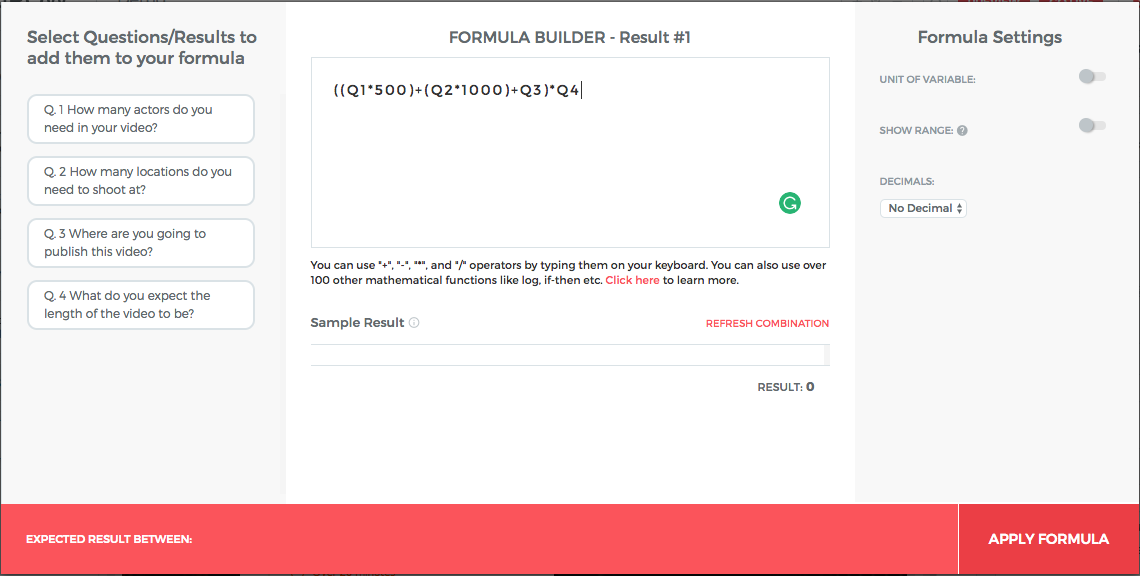# Advanced & Scientific Formulas

You can use basic operators like +, -, x, and / by typing them out on the keyboard. You can easily build simple cost and savings calculators with these basic operators. You can also use advanced operators like ^ and log. Simply type them out on your keyboard.

To understand how to design more complicated formulas, please go through the sections below.

## Using If-Then-Else Statements

To use conditional expressions, you need to use a formula of type ((x) ? y : z). Let's use it in an example for better understanding:
Say, we are trying to assess the risk of you hitting your head on the ceiling and it depends on your height. So you will ask a question "What is your height?" and on the basis of the answer assign a risk value.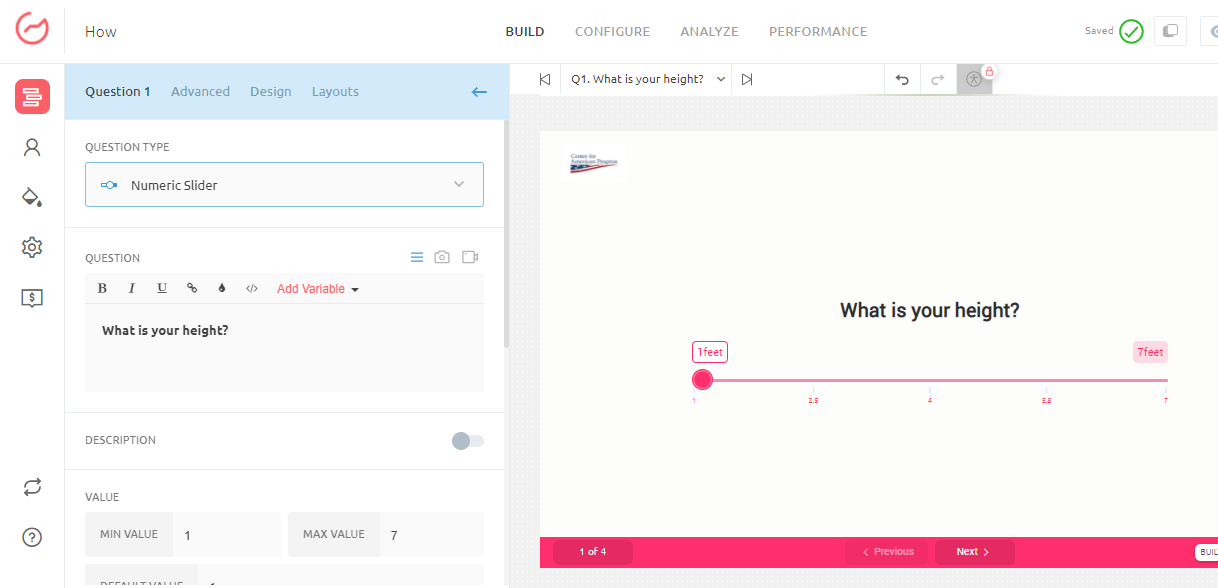The expression will take the form: If my height is < 4.5 feet then my risk of hitting my head on the roof is 50%, else it is 90%. Here's how you can write this expression in the formula builder:

((Q1 < 4.5)? 50 : 90)
*Here, "?" becomes the expression for "then", and ":" becomes the expression for "else".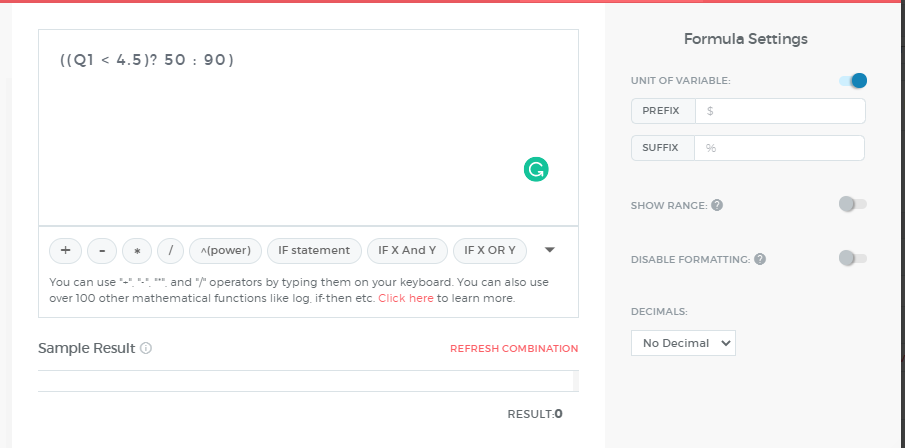## Using Else-If Statements

This was a simple example but it can be more complicated in a real situation. Say, there was another question that asked if you have good eyesight. [if eyesight is good, then we assign a value of 1, else 0]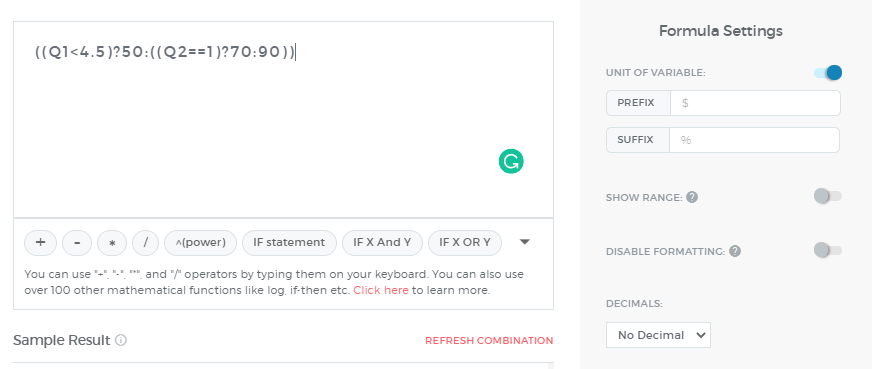If Else Statements in the Outgrow Builder using "OR" and "AND" Keywords:**

To use If Else statements the basic syntax is "((x>0)? y:z)". This says if X is greater than 0, show Y otherwise show Z. Let's use it using an example for "or" and "and" statement for better understanding:

Suppose there that for all values of Q1 (value of question 1 is contained in Q1) less than 0 or greater than 10 the Result (R1) is 0. The solution for this one would be:
(((Q1<0)or(Q1>10))?0:0)

This handles the two ends of the ranges on the far left and far right.

Now let's assume for Q1 between 0 and 5, R1 (result 1) is fixed at 20. The solution for this one would be:
(((Q1>=0)and(Q1<=5))?20:0)

Now when Q1 is between 5 and 10, R1 goes down linearly from 20 to 0. So imagine the value of R1 at 20 when Q1 is 5, at 0 when is 10 and then at Q1 is 7.5, R1 should be at the midpoint so 10. The reason it is in the midpoint is that it goes down linearly.

So, over 5 points of Q1 Result 1 goes down 20. Thus, for every one-point change in "X" from 5, "Y" goes down by 20/5 (or 4). So 4 points reduction for every (Q1-5) point, we can restate this to say that R1 goes down from 20 by the amount 4*(Q1-5). This is the reduction amount from 20, hence, the formula for R1 for Q1 between 5 and 10 would be "20-4x(Q1-5)". Thus, the solution for this would be:

(((Q1>5)and(Q1<=10))?(20-((Q1-5)x4)):0)

## HOW TO HANDLE "IN BETWEEN" SCENARIO:

To handle "in-between" conditions, we should always use greater than (>) and less than (<) symbols and make sure to be careful that the same endpoint is not included in the two conditions.

For example, Income, if income from X to Y has tax of 10% and income from Y to Z has tax of 20%. Y cannot be part of both ranges, so Y must either be 10% or 20%. In this case, let's assume Y is 20%, then we must not include Y in the first range, we do this by using the strictly less than (<) symbol for the first range and then (>=) symbol in the second range.

Here is an example assume Q2 is the question asking a user for his/her income (notice Y is only in the second condition below):
(((Q2>=X)and(Q2<Y))?10:0)+(((Q2>=Y)and(Q2<=Z))?20:0)
__-

## HOW TO USE PARENTHESES:

1. For "and" and "or" statements we always have at least two conditions before a question mark (?). It's important to write both conditions in separate parentheses so that the if statement knows there are two conditions and not just one.

Let us consider this example - do you know what is wrong with this writing here: ((Q1<20)and(Q1>10)?5:0)

What appears before the question mark is (Q1>10). But we don't want to say If Q1>10. We want to say if Q1 is > 10 and Q1 < 20

So we need to put that in parentheses for both conditions so the if statement knows the condition is not just Q1>10.

So the correct format is: (((Q1<20)and(Q1>10))?5:0).

1. Using Parentheses with the simple if-else statement: Since there is only one condition in a simple if-else statement, then only one set of parentheses is required for that condition.

For Example: Let's say if Q1 is 0 then the output should be 10+Q1 else 0.
((Q1==0)?(10+Q1):0)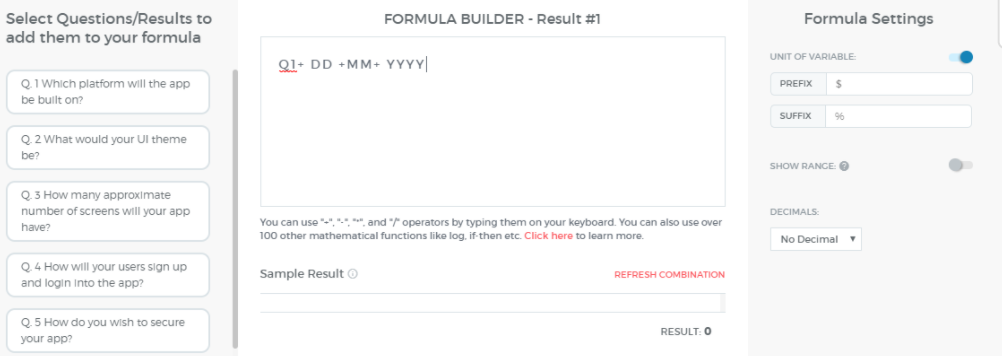### 📘Use date variables in formula

Now you can use date variables in Formulas on your calculator -
Use DD for the current date
Use MM for the current month
and Use YYYY for the current year

Other Operators
For other advanced operators, please see the documentation for operators here and functions here.

Feel free to email [email protected] if you need any help with the formula. We will be delighted to help :)